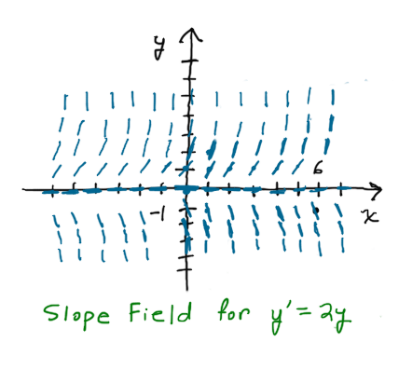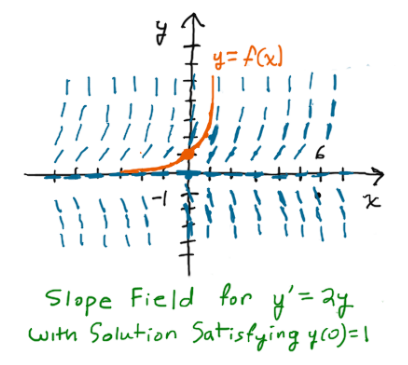# Example: How to Make and Interpret a Basic Slope Field

1. Sketch the slope field for the differential equation y'=2y.
2. Sketch the graph of the solution of y'=2y satisfying y(0)=1.
3. What do we call the graph of a solution to a differential equation?